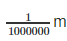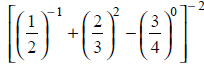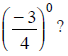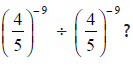Courses

# Exponents And Powers - MCQ

## 10 Questions MCQ Test Class 8 Mathematics by VP Classes | Exponents And Powers - MCQ

Description
This mock test of Exponents And Powers - MCQ for Class 8 helps you for every Class 8 entrance exam. This contains 10 Multiple Choice Questions for Class 8 Exponents And Powers - MCQ (mcq) to study with solutions a complete question bank. The solved questions answers in this Exponents And Powers - MCQ quiz give you a good mix of easy questions and tough questions. Class 8 students definitely take this Exponents And Powers - MCQ exercise for a better result in the exam. You can find other Exponents And Powers - MCQ extra questions, long questions & short questions for Class 8 on EduRev as well by searching above.
QUESTION: 1

Solution:
QUESTION: 2

Solution:
QUESTION: 3

### Which of the following is the standard form of 0.00001275?

Solution:
QUESTION: 4

Which of the following is the used form of 5 . 05 × 106 ?

Solution:
QUESTION: 5

For which of the following m = 8?

Solution:
QUESTION: 6

1 micron is equal to. Which of the following is its standard form?

Solution:
QUESTION: 7is equal to:

Solution:
QUESTION: 8

Which of the following is equal to [100 – 990] × 100?

Solution:
QUESTION: 9

Which of the following is the reciprocal ofSolution:
QUESTION: 10

Which of the following is the value ofSolution: